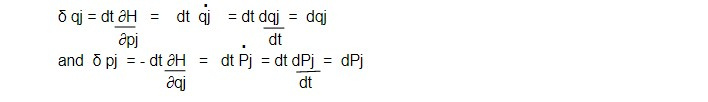# Poisson's Second Theorem

Statement: '' The poisson bracket of two constant of motion is itself a constant of motion.''

If u and v are constant of motions then their poisson's bracket is also constant of motion. i.e

[u, v ] = constant

According to Jacobi's identity

[x, [y, z]] + [y, [z,x]] + [z, [x,y]] =0 .......1

Let x -> H, y->u and z ->v, then 1 becomes

[H, [u, v]] + [u, [v,H]] + [V, [H,u]] =0......2

Since u and v are constant function of motion . From equation 2 and 3, we get

[u, H] = 0

=> [v, H] = 0 .......3

where u and v are not explicit function of time. From equation 2 and 3, we get

[ H, [u,v]] = 0

=> [ [u,v], H] = 0......4

Again equation of motion in poisson bracket form for a dynamical variable F(qj, p,t) can be expressed as

dF/dt = [F, H] + ∂F/ ∂t

If F doesn't involve time explicitly then

dF/dt = [ F, H] .............5

Also, d [u,v] /dt = [ [u,v],H] =0.......6

Using 4 in 6, we get

[u,v] = constant

General case:

If u and v contain explicit function of time, then

du/dt = [u,H] + ∂u/∂t

or, 0 = [u,H] + ∂u/∂t

=> ∂u/∂t = [u,H] = [H,u]

Similarly ∂v/∂t = [H,v] ......7

Using the relation in equation 2, we get

[H, [u,v]] - [u, ∂v/∂t ]- [∂u/∂t , v] =0

or, [H, [u,v]] + [ ∂v/∂t , u] + [v, ∂u/∂t ] =0

or, [H, [u,v]] - ∂ [u,v]/∂t =0

or, [ [u,v] , H] + ∂ [u,v] /∂t = 0

or, d [u,v]/dt = 0

=> [u,v] = constant

Infinitesimal contact /cannonical transformation

The transformation in which old and new coordinates are differ slightly is known as infinitesimal contact or cannonical transformation.

Let us take a generating function of type

F2 = Σ qjPj ....1

Then we have, Qj = ∂F2/ ∂Pj = qj

=> Qj = qj

And pj = ∂F2/ ∂qj = Pj

=> pj = ∂F2/∂qj = Pj

=> Pj = pj

This type of transformation is called identity transformation.

If ∊ be an infinitesimal parameter independent of qj and Pj then

F = qjPj + ∊G (qj,Pj) will generate an infinitesimal change in the variable, where G is any arbitrary function.

The new variables are given as

Qj = ∂F/∂Pj = qj + ∊ ∂G (qj, Pj)/ ∂Pj

and pj = ∂F/∂qj = Pj + ∊ ∂G (qj, Pj)/ ∂qj

So that

δ qj = Qj - qj = ∊ ∂G (qj, Pj)/ ∂Pj

δpj = Pj - pj = - ∊ ∂G (qj, Pj)/ ∂qj

Here G acts as the generating function of the infinitesimal contact or cannonical transformation.

Consider an infinitesimal cannonical transformation in which G is the Hamiltonian H(q,p) and ∊ is an infinitesimal interval of time dt, thenThey represent an infinitesimal change in the coordinates and momenta when the system moves for a small time 'dt'.

These equations represent a transformation from the variables qj,pj at time t to the variables qj+ δqj, pj+ δpj at time t+dt can be described by an infinitesimal transformation generated by the Hamiltonian. Hence, we can state that the Hamiltonian is the generator for the motion of the system with time.

This note is taken from Classical Mechanics, MSC physics, Nepal.

This note is a part of the Physics Repository.